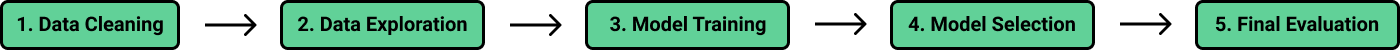# Lesson: Introduction to Machine Learning Concepts

Machines do not learn information the same way that we humans do. While humans are better at learning general concepts, computers are more adept at learning how to perform highly specific tasks. We supply a computer with data so that it can try to derive useful patterns from the data itself. This process of extracting patterns from data is what we call machine learning.

Throughout this course, we'll learn about the process of machine learning and how to perform it through the example of an algorithm called the k-nearest neighbors algorithm. We'll call this process the machine learning workflow. We call it a workflow, because it is a general process that people can follow to perform many basic machine learning tasks.The k-nearest neighbors algorithm is just one of many machine learning algorithms, but the workflow is algorithm-agnostic, meaning that it can be used no matter what the algorithm. Learning this workflow first will help make learning and using more algorithms easier in the future. Before we dive further into the learning process, let's get familiar with the data set we'll be working with.

#### Objectives

• The basics of the machine learning workflow.
• How the k-nearest neighbors algorithm works.

#### Lesson Outline

1. Introduction to Machine Learning
2. Exploring the Airbnb data set
3. The K-nearest neighbors algorithm
4. A numerical description for similarity
5. Calculate distance for all observations
6. Using randomization
7. Cleaning the price column
8. Calculating average price
9. Next steps
10. Takeaways